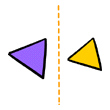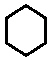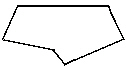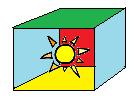Name:    2nd Grade Geometry - Reason with shapes and their attrributes, Standard 1, Post Test 1 Recognize and draw shapes having specified attributes, such as a given number of angles or a given number of equal faces. Identify triangles, quadrilaterals, pentagons, hexagons, and cubes.

Multiple Choice
Identify the choice that best completes the statement or answers the question.

1.

Suzie had a triangle-shaped piece of colored paper.  She folded the top over in a straight line to make a new shape.  What shape does she now have?
 a. hexagon c. triangle b. quadrilateral d. pentagon

2.

If Suzie takes her triangle and cuts off the two lower corners instead of the top, what shape would she have?
 a. hexagon c. cube b. rectangle d. pentagon

3.

Suzie folded her paper one more time and came up with a shape that had six angles.  What shape did she have now?
 a. pentagon c. unilateral b. hexagon d. cube

4.How do we know these are triangles??
 a. they look like triangles c. they have three angles b. they look like pyramids d. none of the above

5.Tony put all these squares together to form a box.  What shape did he now have?
 a. cube c. square b. pentagon d. quadrilateral

6.The home plate in baseball looks like this.  What shape is it??
 a. cube c. square b. pentagon d. hexagon

7.Which shape is this?
 a. cube c. square b. pentagon d. hexagon

8.

Mary drew a shape on her paper like this.  When she was done, she said, “I just drew a _____!”
 a. unilateral c. quadrilateral b. pentagon d. hexagon

9.

What type of shape is the sun in?a. square c. cube b. quadrilateral d. box

10.

Out of which shape do we make pyramids?
 a. pentagon c. hexagon b. quadrilateral d. triangles

Numeric Response

11.

Wendy counted the angles in a hexagon.  How many did she count?

12.

Bobby started counting the angles on a quadrilateral shape.  How many did he count?